# insertObjectAnnotation

Annotate truecolor or grayscale image or video stream

## Syntax

``RGB = insertObjectAnnotation(I,shape,position,label)``
``insertObjectAnnotation(I,"rectangle",position,label)``
``insertObjectAnnotation(I,"circle",position,label)``
``___ = insertObjectAnnotation(___,Name=Value)``

## Description

example

````RGB = insertObjectAnnotation(I,shape,position,label)` returns a truecolor image annotated with `shape` and `label` at the location specified by `position`.```

example

````insertObjectAnnotation(I,"rectangle",position,label)` inserts rectangles and labels at the location indicated by the position matrix.```
````insertObjectAnnotation(I,"circle",position,label)` inserts circles and corresponding labels at the location indicated by the position matrix.```

example

````___ = insertObjectAnnotation(___,Name=Value)` specifies options using one or more name-value arguments in addition to the previous syntax. For example,`insertObjectAnnotation(I,"rectangle",position,label_str,FontSize=18)` sets the annotation font size to `18`.```

## Examples

collapse all

`I = imread("board.tif");`

Create labels of floating point numbers. The floating point numbers relate to confidence value labels.

```label_str = cell(3,1); conf_val = [85.212 98.76 78.342]; for ii=1:3 label_str{ii} = ['Confidence: ' num2str(conf_val(ii),'%0.2f') '%']; end```

Set the position for the rectangles in the form of [x y width height].

`position = [23 373 60 66;35 185 77 81;77 107 59 26];`

Insert the labels.

`RGB = insertObjectAnnotation(I,"rectangle",position,label_str,TextBoxOpacity=0.9,FontSize=18);`

Display the annotated image.

```figure imshow(RGB) title("Annotated chips");```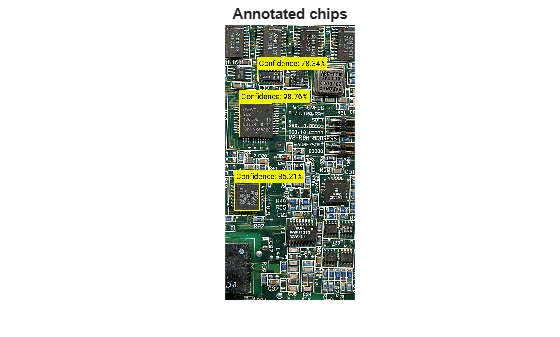Read an image into the workspace.

`I = imread("coins.png");`

Set the positions on the image for the circle shapes. The first two values represents the center at (x,y) and the third value is the radius.

`position = [96 146 31;236 173 26];`

Set the label to display the integers 5 and 10 (U.S. cents).

`label = [5 10];`

Insert the annotations.

`RGB = insertObjectAnnotation(I,"circle",position,label,LineWidth=3,Color=["cyan","yellow"],TextColor="black");`

Display.

```figure imshow(RGB) title("Annotated coins");```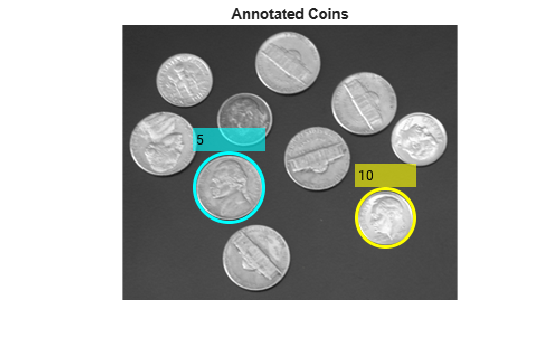## Input Arguments

collapse all

Truecolor or grayscale image, specified as an image or video stream. The input image can be either an M-by-N-by-3 truecolor or a M-by-N 2-D grayscale image.

Data Types: `double` | `single` | `uint8` | `uint16` | `int16`

Shape of annotation, specified as `"rectangle"` or `"circle"`

Data Types: `char`

Position of shape, specified according to the type of shape, described in the table.

ShapePositionExample
`"rectangle"`For one or more rectangles, specify M-by-4 matrix where each row specifies a rectangle as $\left[\begin{array}{cccc}x& y& width& height\end{array}\right]$.

`$\left[\begin{array}{cccc}{x}_{1}& {y}_{1}& widt{h}_{1}& heigh{t}_{1}\\ {x}_{2}& {y}_{2}& widt{h}_{2}& heigh{t}_{2}\\ ⋮& ⋮& ⋮& ⋮\\ {x}_{M}& {y}_{M}& widt{h}_{M}& heigh{t}_{M}\end{array}\right]$`

Two rectangles, M=2`"circle"`An M-by-3 matrix, where each row is a vector specifying a circle as $\left[\begin{array}{ccc}x& y& radius\end{array}\right]$. The $\left[\begin{array}{cc}x& y\end{array}\right]$ coordinates represent the center of the circle.

`$\left[\begin{array}{ccc}{x}_{1}& {y}_{1}& radiu{s}_{1}\\ {x}_{2}& {y}_{2}& radiu{s}_{2}\\ ⋮& ⋮& ⋮\\ {x}_{M}& {y}_{M}& radiu{s}_{M}\end{array}\right]$``"projected-cuboid"`

An 8-by-2-by-M array or an M-by-8 matrix, where M specifies a projected cuboid.

When specified as an 8-by-2-M array, each row must contain the $\left[\begin{array}{cc}x& y\end{array}\right]$ location of a projected cuboid vertex. The vertices are connected to form a cuboid with six faces. The order of the input vertices must match the order shown in the diagram.

When specified as an M-by-8 matrix, each row specifies the front-facing and rear-facing sides of a projected cuboid in the form,

`$\left[\begin{array}{cccccccc}x1& y1& w1& h1& x2& y2& w2& h2\end{array}\right]$`

where, [x1 y1] and [x2 y2] specify the upper-left coordinates of the front-facing and back-facing sides, respectively. [w1 h1] and [w2 h2] specify the corresponding width and height.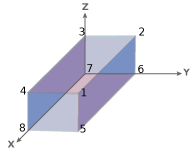Data Types: `single` | `double` | `int8` | `int16` | `int32` | `int64` | `uint8` | `uint16` | `uint32` | `uint64`

Label to associate with a shape, specified as an M numeric vector, string, categorical labels, or a cell array of ASCII character vectors. The character, string, or categorical labels must be encoded as ASCII characters. The cell array must be the of length equal to the number of shape positions. You can specify a scalar label for all shapes as a numeric scalar, string, or categorical.

Example: label = [5 10], where the function marks the first shape with the label, 5, and the second shape with the label, 10.

### Name-Value Arguments

Specify optional pairs of arguments as `Name1=Value1,...,NameN=ValueN`, where `Name` is the argument name and `Value` is the corresponding value. Name-value arguments must appear after other arguments, but the order of the pairs does not matter.

Before R2021a, use commas to separate each name and value, and enclose `Name` in quotes.

Example: `Color="white"` sets the color for the label text box to white.

Font face of text, specified as a character vector. The font face must be one of the available truetype fonts installed on your system. To get a list of available fonts on your system, type `listTrueTypeFonts` at the MATLAB® command prompt.

Data Types: `char`

Label text font size, specified as an integer that corresponds to points in the range of [`8 72`].

Data Types: `double` | `single` | `int8` | `int16` | `int32` | `int64` | `uint8` | `uint16` | `uint32` | `uint64`

Shape border line width, specified as a positive scalar integer in pixels.

Shape color, specified as a character vector, cell array of character vectors, vector, or an M-by-3 matrix. You can specify a different color for each shape or one color for all shapes. Color values must be specified in the range `[0,255]`. Values that have a range of `[0,1]` must be scaled by a value of 255 before using it with this function. For example, `[255 255 255].*colorvalue`.

Supported colors are: `"blue"`, `"green"`, `"red"`, `"cyan"`, `"magenta"`, `"yellow"`,`"black"`, and `"white"`.

ColorFormatExample
Specify one color for all shapes

String or character color name

`"r"`

`"red"`

1-by-3 vector (RGB triplet)

`[255 0 0]`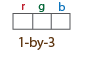Specify a color for each shapeM-element vector

`["red","yellow","blue"]`

M-by-3 matrix, as a list of RGB values

```255 0 0 255 0 0 0 255 255```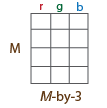Data Types: `cell` | `char` | `uint8` | `uint16` | `int16` | `double` | `single`

Color of text in text label, specified as a character vector, an [R G B] vector, a cell array, or an M-by-3 matrix. To specify one color for all text, set this parameter to either a character vector or an [R G B] vector. To specify a color for each of the M text labels, set this parameter to a cell array of M character vectors. Alternatively, you can specify an M-by-3 matrix of RGB values for each annotation. RGB values must be in the range of the input image data type.

Supported colors: `"blue"`, `"yellow"`,`"green"`, `"cyan"`, `"red"`, `"magenta"`, `"black"`, and `"white"`.

Data Types: `char` | `uint8` | `uint16` | `int16` | `double` | `single` | `cell`

Opacity of text label box background, specified as a scalar defining the opacity of the background of the label text box. Specify this value in the range of `0` to `1`.

Data Types: `double` | `single` | `int8` | `int16` | `int32` | `int64` | `uint8` | `uint16` | `uint32` | `uint64`

## Output Arguments

collapse all

Truecolor image with annotation, returned as an image or video stream.

Data Types: `double` | `single` | `uint8` | `uint16` | `int16`

## Version History

Introduced in R2012b

expand all